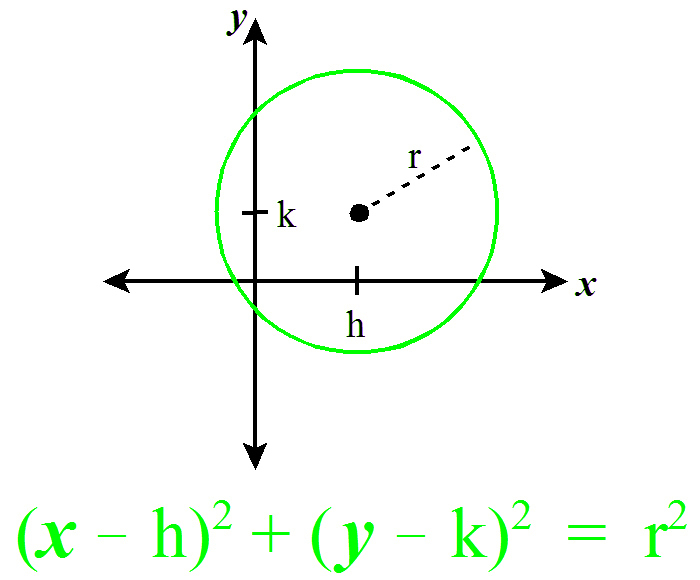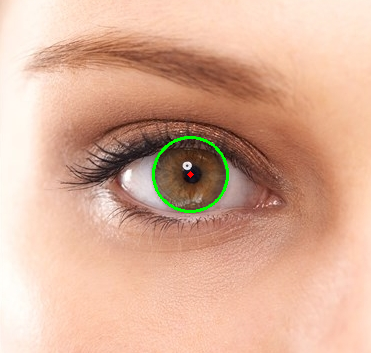# Circle Detection using OpenCV | Python

Circle detection finds a variety of uses in biomedical applications, ranging from iris detection to white blood cell segmentation. The technique followed is similar to the one used to detect lines, as discussed in this article.

Basics of Circle Detection

A circle can be described by the following equation:To detect circles, we may fix a point (x, y). Now, we are required to find 3 parameters: a, b and r. Therefore, the problem is in a 3-dimensional search space. To find possible circles, the algorithm uses a 3-D matrix called the “Accumulator Matrix” to store potential a, b and r values. The value of a (x-coordinate of the center) may range from 1 to rows, b (y-coordinate of the center) may range from 1 to cols, and r may range from 1 to maxRadius =.

Below are the steps of the algorithm.

• Initializing the Accumulator Matrix: Initialize a matrix of dimensions rows * cols * maxRadius with zeros.
• Pre-processing the image: Apply blurring, grayscale and an edge detector on the image. This is done to ensure the circles show as darkened image edges.
• Looping through the points: Pick a pointon the image.
• Fixing r and looping through a and b: Use a double nested loop to find a value of r, varying a and b in the given ranges.

 for a in range(rows):      for b in range(cols):          r = math.sqrt((xi - a)**2 + (yi - b)**2)          accum_matrix[a][b][r] += 1

• Voting: Pick the points in the accumulator matrix with the maximum value. These are strong points which indicate the existence of a circle with a, b and r parameters. This gives us the Hough space of circles.
• Finding Circles: Finally, using the above circles as candidate circles, vote according to the image. The maximum voted circle in the accumulator matrix gives us the circle.

The HoughCircles function in OpenCV has the following parameters which can be altered according to the image.

Detection Method: OpenCV has an advanced implementation, HOUGH_GRADIENT, which uses gradient of the edges instead of filling up the entire 3D accumulator matrix, thereby speeding up the process.
dp: This is the ratio of the resolution of original image to the accumulator matrix.
minDist: This parameter controls the minimum distance between detected circles.
Param1: Canny edge detection requires two parameters — minVal and maxVal. Param1 is the higher threshold of the two. The second one is set as Param1/2.
Param2: This is the accumulator threshold for the candidate detected circles. By increasing this threshold value, we can ensure that only the best circles, corresponding to larger accumulator values, are returned.

Below is the code for finding circles using OpenCV on the above input image.

 import cv2  import numpy as np     # Read image.  img = cv2.imread('eyes.jpg', cv2.IMREAD_COLOR)     # Convert to grayscale.  gray = cv2.cvtColor(img, cv2.COLOR_BGR2GRAY)     # Blur using 3 * 3 kernel.  gray_blurred = cv2.blur(gray, (3, 3))     # Apply Hough transform on the blurred image.  detected_circles = cv2.HoughCircles(gray_blurred,                      cv2.HOUGH_GRADIENT, 1, 20, param1 = 50,                 param2 = 30, minRadius = 1, maxRadius = 40)     # Draw circles that are detected.  if detected_circles is not None:         # Convert the circle parameters a, b and r to integers.      detected_circles = np.uint16(np.around(detected_circles))         for pt in detected_circles[0, :]:          a, b, r = pt, pt, pt             # Draw the circumference of the circle.          cv2.circle(img, (a, b), r, (0, 255, 0), 2)             # Draw a small circle (of radius 1) to show the center.          cv2.circle(img, (a, b), 1, (0, 0, 255), 3)          cv2.imshow("Detected Circle", img)          cv2.waitKey(0)

Output:My Personal Notes arrow_drop_upCheck out this Author's contributed articles.

If you like GeeksforGeeks and would like to contribute, you can also write an article using contribute.geeksforgeeks.org or mail your article to contribute@geeksforgeeks.org. See your article appearing on the GeeksforGeeks main page and help other Geeks.

Please Improve this article if you find anything incorrect by clicking on the "Improve Article" button below.

Article Tags :

2

Please write to us at contribute@geeksforgeeks.org to report any issue with the above content.